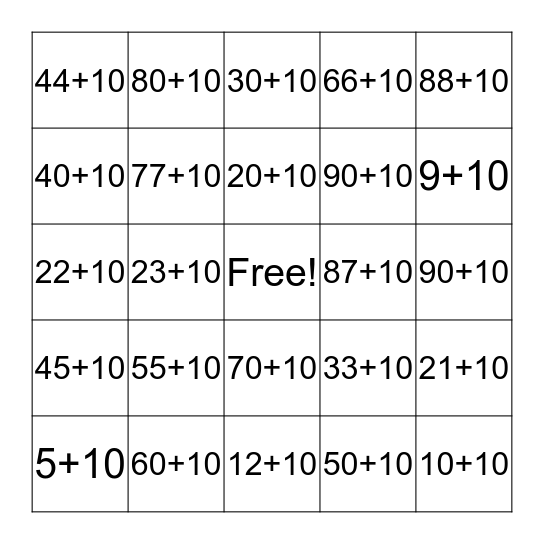This bingo card has a free space and 24 words: 55+10, 60+10, 20+10, 5+10, 88+10, 44+10, 40+10, 80+10, 87+10, 70+10, 90+10, 22+10, 33+10, 21+10, 77+10, 50+10, 90+10, 30+10, 9+10, 23+10, 10+10, 45+10, 66+10 and 12+10.

⚠ This card has duplicate items: 90+10 (2)

## Play Online